﻿ 地电场观测方法与观测技术研究文章快速检索
 地震地磁观测与研究2019, Vol. 40Issue (2): 1-20  DOI: 10.3969/j.issn.1003-3246.2019.02.0010

### 引用本文Xi Jilou. A review of geoelectric field observation methods and techniques[J]. Seismological and Geomagnetic Observation and Research, 2019, 40(2): 1-20. DOI: 10.3969/j.issn.1003-3246.2019.02.001.### 文章历史

1. 中国北京 100036 中国地震局地震预测研究所;
2. 中国北京 100036 中国地震局地震预测重点实验室

A review of geoelectric field observation methods and techniques
1. Institute of Earthquake Forecasting, China Earthquake Administration, Beijing 100036, China;
2. Key Laboratory of Earthquake Prediction, China Earthquake Administration, Beijing 100036, China
Abstract: Geoelectric field is an important geophysical field. The natural electric field on the surface of the earth and its change with time are the main research objects, and it is an important part of the geomagnetic field. On the basis of a brief analysis of the current situation of the geoelectric field observation and research at home and abroad, combined with the practice of the geoelectric field observation in China for more than 20 years, this paper systematically analyses and summarizes the research progress in the main aspects of geoelectric field observation objects, observation methods, observation techniques, observation data and so on. And at the same time, the restricts of its future development, the main factors of the exhibition, as well as some issues that need to be focused on at present, are preliminarily analyzed and discussed in this paper.
Key words: geoelectric field    observation method    observation technology    observation data    review
0 引言

20世纪90年代开始，我国地震部门的领导和专家，以专项考察、交流访问、资料翻译、分析和研究等形式，对希腊VAN方法予以关注和重视。在此基础上，通过国际合作、自主研发和探索研究等途径，利用十多年时间，初步建设完成覆盖我国大陆地区各主要构造带、活动断裂带、地震危险区和重点监视区的地电场观测台网，开启了我国数字化地电场观测与应用研究工作（席继楼等，2016）。

1 地电场观测对象

2 地电场观测方法 2.1 地电场基本观测原理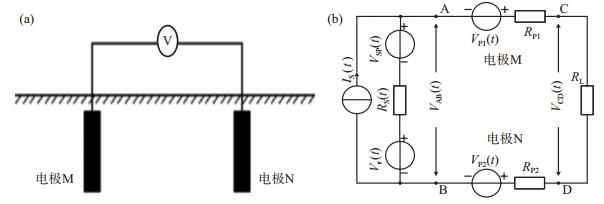图 1 地电场观测基本原理示意 (a)基本原理；(b)等效模型 Fig.1 Sketch of the basic principle of the geoelectric field observation
 $E_{\mathrm{MN}}=-\frac{\Delta U_{\mathrm{MN}}}{L_{\mathrm{MN}}}$ (1)

 $V_{\mathrm{AB}}(t)=R_{\mathrm{S}}(t) \cdot I_{\mathrm{S}}(t)+V_{\mathrm{SP}}(t)+V_{\mathrm{r}}(t)$ (2)
 $V_{\mathrm{CD}}(t)=\frac{\left(R_{\mathrm{P} 1}+R_{\mathrm{P} 2}+R_{\mathrm{L}}\right) \cdot R_{\mathrm{S}}(t)}{R_{\mathrm{P} 1}+R_{\mathrm{P} 2}+R_{\mathrm{L}}+R_{\mathrm{S}}(t)} \cdot I_{\mathrm{S}}(t)+\frac{R_{\mathrm{L}}\left\{V_{\mathrm{SP}}(t)+V_{\mathrm{r}}(t)+\left[V_{\mathrm{Pl}}(t)-V_{\mathrm{P} 2}(t)\right]\right\}}{R_{\mathrm{P} 1}+R_{\mathrm{P} 2}+R_{\mathrm{L}}+R_{\mathrm{S}}(t)}$ (3)

 $V_{\mathrm{CD}}(t) \approx R_{\mathrm{S}}(t) \cdot I_{\mathrm{S}}(t)+V_{\mathrm{SP}}(t)+V_{\mathrm{r}}(t)+\left[V_{\mathrm{P} 1}(t)-V_{\mathrm{P} 2}(t)\right]$ (4)

 $V_{\mathrm{CD}}(t) \approx R_{\mathrm{S}}(t) \cdot I_{\mathrm{S}}(t)+V_{\mathrm{SP}}(t)+V_{\mathrm{r}}(t)=V_{\mathrm{AB}}(t)$ (5)

 $V_{\mathrm{AB}}(t) \approx R_{\mathrm{S}}(t) \cdot I_{\mathrm{S}}(t)+V_{\mathrm{SP}}(t)$ (6)

2.2 自然电场观测方法

 $E_{\mathrm{SP}} \approx \overline{E}_{\mathrm{MN}}=\frac{1}{1440} \sum\limits_{k=0}^{1439}\left(E_{\mathrm{MN}}\right)_{k}$ (7)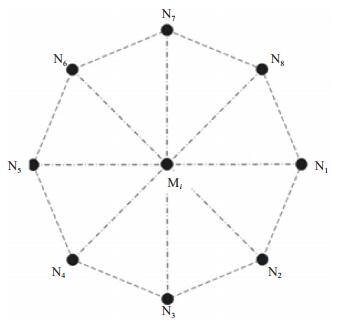图 2 自然电场多方位观测方法示意 Fig.2 The method of the multi-directional observation of the spontaneous potential
 $V_{\mathrm{SP}}=\frac{1}{N} \sum\limits_{k=0}^{N-1}\left(V_{\mathrm{MN}}\right)_{k}$ (8)
 $E_{\mathrm{SP}}=\frac{1}{N} \sum\limits_{k=0}^{N-1}\left(E_{\mathrm{MN}}\right)_{k}$ (9)
2.3 大地电场观测方法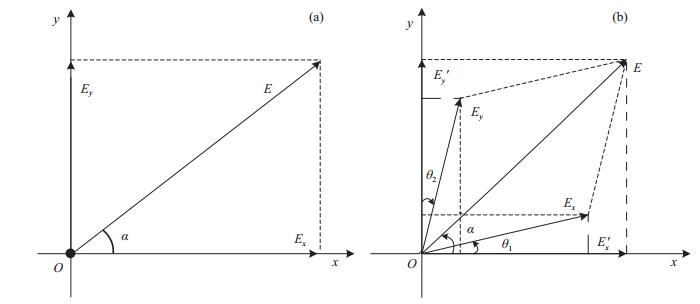图 3 大地电场矢量观测方法示意 (a)正交分量Ex和Ey观测；(b)非正交分量Ex和Ey观测 Fig.3 The sketch of the method of the vector observation of the telluric field
 $E_{\mathrm{T}}=\left(E_{\mathrm{MN}}\right)_{0}-E_{\mathrm{SP}}$ (10)
 $E=\sqrt{E_{x}^{2}+E_{y}^{2}}$ (11)
 $\alpha=\operatorname{tg}^{-1} \frac{E_{y}}{E_{x}}$ (12)

 $E_{x}^{\prime}=E_{x} \cos \theta_{1}+E_{y} \sin \theta_{2}$ (13)
 $E_{y}^{\prime}=E_{x} \sin \theta_{1}+E_{y} \cos \theta_{2}$ (14)
 $E=\sqrt{E_{x}^{2}+E_{y}^{2}+2 E_{x} E_{y} \sin \left(\theta_{1}+\theta_{2}\right)}$ (15)
 ${\rm{ \mathsf{ α} }} = {\rm{t}}{{\rm{g}}^{ - 1}}\frac{{{E_x}\sin {\theta _1} + {E_y}\cos {\theta _2}}}{{{E_x}\cos {\theta _1} + {E_y}\sin {\theta _2}}}$ (16)
2.3 地电场多装置观测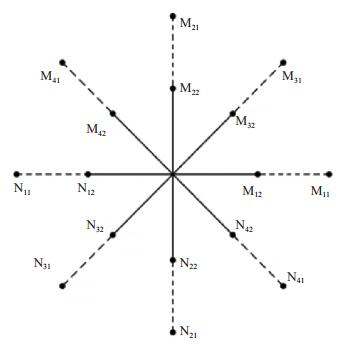图 4 地电场多装置观测方法示意 Fig.4 The sketch of the method of the multi-device observation of the geoelectric field

 ${r_{xy}} = \frac{{\sum\limits_{i = 0}^{N - 1} {\left({{y_i} - \bar y} \right)} \left({{x_i} - \bar x} \right)}}{{\sqrt {\sum\limits_{i = 0}^{N - 1} {{{\left({{y_i} - \bar y} \right)}^2}} } \cdot \sqrt {\sum\limits_{i = 0}^{N - 1} {{{\left({{x_i} - \bar x} \right)}^2}} } }}$ (17)

 ${\mathit{\Delta }_{xy}} = \frac{{\sum\limits_{i = 0}^{N - 1} {\left[ {\left({{x_i} - {x_d}} \right) - K \cdot \left({{y_i} - {y_d}} \right)} \right]} }}{N}$ (18)

3 地电场观测技术 3.1 测量电极

3.2 地电场观测仪器

2000年以后，为了适应我国地震前兆观测网络的发展需求，在原ZD9A地电场仪观测原理基础上，开展了基于互联网数据传输与控制技术的改型研究，主要研究成果包括ZD9A-Ⅱ地电场仪、ZD9A-2B地电场仪和ZD9A-SP地电场仪等。此类仪器的共同特点是，均采用如图 5所示的分布式双CPU结构，并利用高级语言编写的多任务/多线程工作程序，完成地电场信号的采集处理和地电场观测数据的网络通信、传输和控制等功能，可实现最高每秒钟一次的多测道地电场同步观测，主要工作流程见图 6。其中，ZD9A-Ⅱ地电场仪采用包含PC104接口的X86+Win98技术构架，实现网络通信、传输和控制功能；ZD9A-2B地电场仪和ZD9A-SP地电场仪采用ARM+WinCE技术构架，实现所有网络功能，并通过综合性技术措施，大幅度提升其环境适应能力（席继楼等，2016）。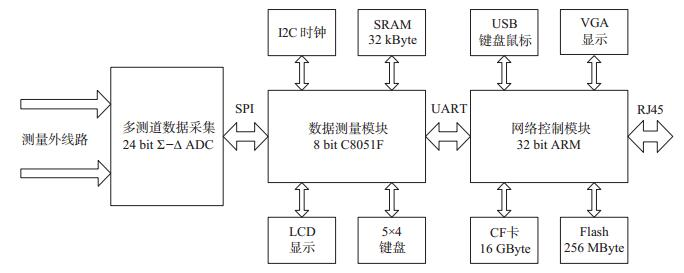图 5 ZD9A-2B地电场仪原理示意 Fig.5 The principle of the ZD9A-2B geoelectric field instrument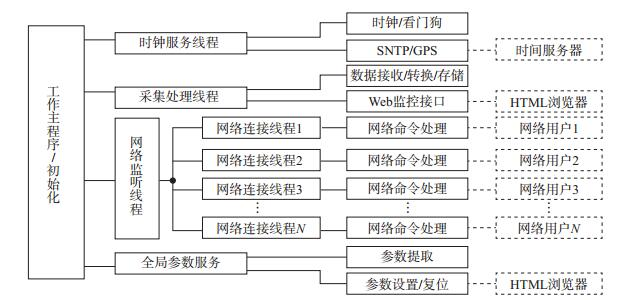图 6 ZD9A-2B地电场仪多任务/多线程工作软件流程 Fig.6 Fig.6 The flow chart of the multi-task/multi-thread software of the ZD9A-2B geoelectric field meter

4 地电场观测数据 4.1 地电场特征变化

4.1.1 地电场静日变化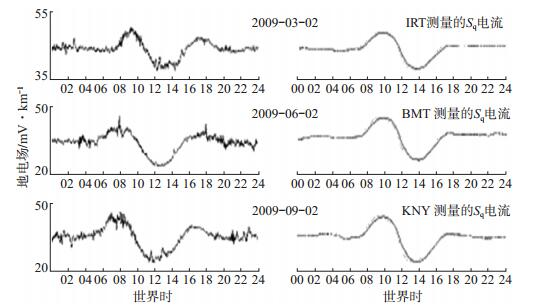图 7 平凉台地电场日变化曲线与Sq电流变化曲线对比分析（据谭大诚等，2010） (a)平凉台地电场NS分量曲线；(b) Sq电流变化曲线 Fig.7 The comparison between the diurnal curve of the geoelectric field and the Sq current variation curve (Tan Dacheng et al, 2010)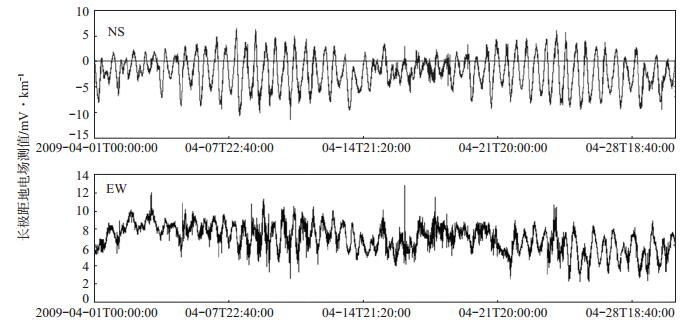图 8 江苏海安台地电场受潮汐影响的地电场数据曲线（据钱家栋等，2010） Fig.8 The curve of the geoelectric field affected by tide from Haian station, Jiangsu Province (Qian Jiadong et al, 2010)
4.1.2 地电暴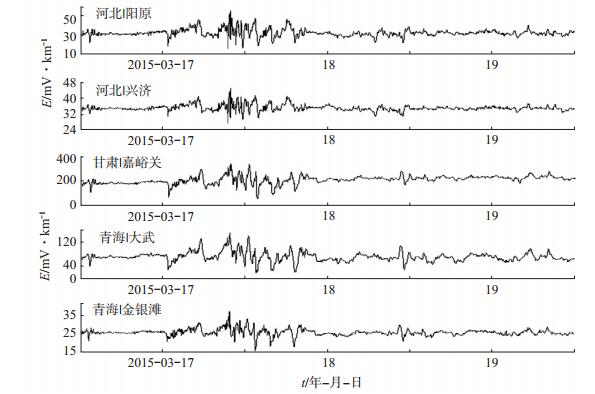图 9 部分台站地电暴同步变化数据曲线 Fig.9 The synchronous variation of the geoelectric storms observated by partial stations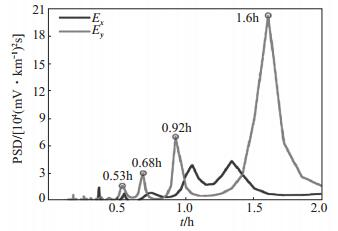图 10 海安地电台2015年6月23日地电暴2 h以下周期频谱（据孙君嵩等（2017）） Fig.10 The periodic spectrum within less than 2 hours of the geoelectric storms observated by the Hai'an station on June 23, 2015 (Sun Junsong et al, 2017)
4.2 典型电磁干扰变化

4.2.1 同场地观测的地电阻率人工供电干扰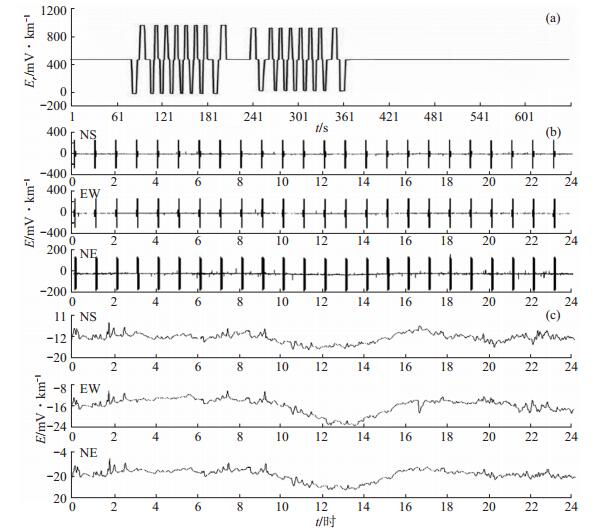图 11 地电阻率人工供电干扰波形及实时处理结果曲线 (a)干扰时序波形；(b)秒采样干扰记录曲线（2015年3月31日）；(c)分钟数据实时处理记录曲线（2015年3月31日） Fig.11 The artificial power supply interference from the geoelectric resistivity observation and the real-time processing result from the geoelectric field observation

4.2.2 地铁/轻轨干扰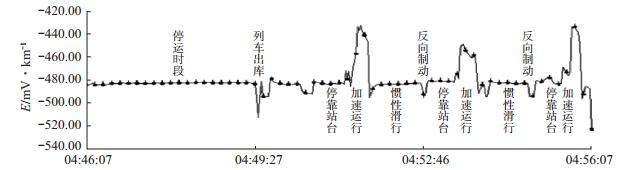图 12 2002年5月16日北京地铁运行漏电干扰波形测试曲线 Fig.12 The curve of leakage interference waveform from Beijing Metro operating on May 16, 2002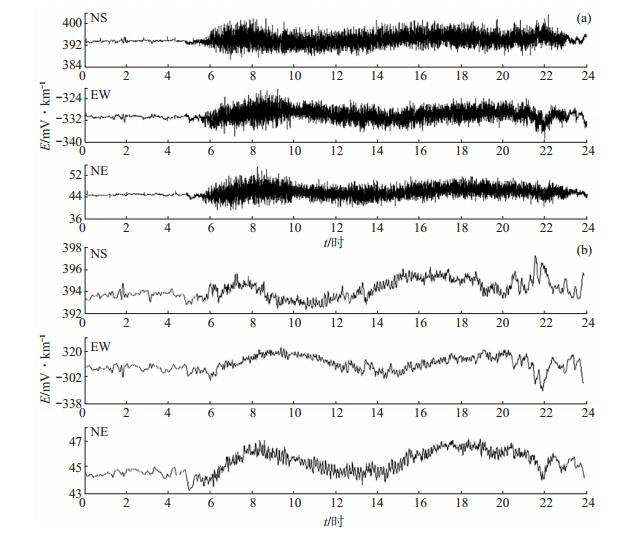图 13 天津地铁运行漏电对天津静海台地电场观测干扰及实时处理曲线 (a)秒采样干扰影响记录曲线；(b)分钟数据实时处理记录曲线 Fig.13 The leakage interference of Tianjin Metro operating and the real-time processing result from the geoelectric field observation at Jinghai station, Tianjin Municipality
4.2.3 超高压直流输电干扰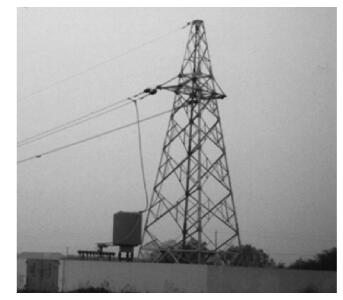图 14 三峡至常州±500 kV直流输电线路常州接地极 Fig.14 The Changzhou ground electrod of the ±500 kV HVDC from Three Gorges to Changzhou

2002年12月，在三峡至常州±500 kV直流输电线路运行测试期间，以常州接地极为起点，沿该直流输电线路的延长线方向布设长100 km的测线，不等间距的布置4个电磁流动观测点，在该输电线路以单极大地回路方式运行时，同步记录接地极附近地区干扰电磁场分布及变化特性。图 15为2002年12月8日记录的一组试验数据，该数据完整记录了输送电流从0 A逐步上升到3 000 A的过程，并清晰记录了线路输送电流从3 000 A突然降回0 A时，各个流动观测点的地电场同步阶跃变化。统计并分析该试验数据，并对每一个测点的试验数据进行线性拟合，结果见图 16。由图 16可见，在每一个测试点，干扰电场的变化幅度与输送电流大小呈线性关系，与接地极的距离呈非线性变化。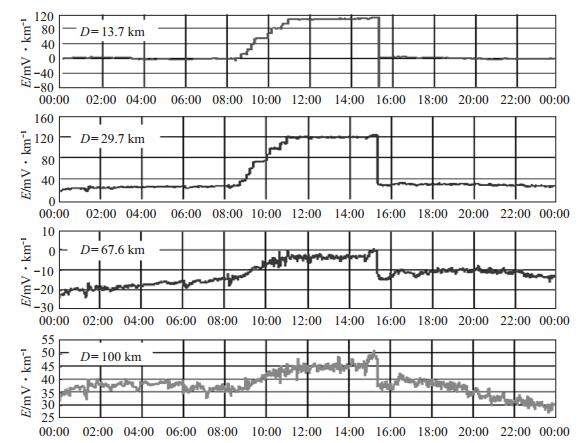图 15 2002年12月8日±500 kV超高压直流输电产生的EW方位干扰电场数据曲线 Fig.15 The EW direction disturbance electric field from the ±500 kV HVDC on Dec.8, 2002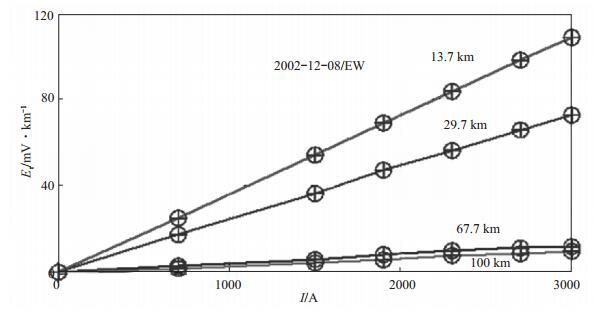图 16 ±500 kV超高压直流输电产生的EW方位干扰电场统计和拟合分析结果 Fig.16 The statistical and fitting analysis result of the EW direction disturbance electric field from the ±500 kV HVDC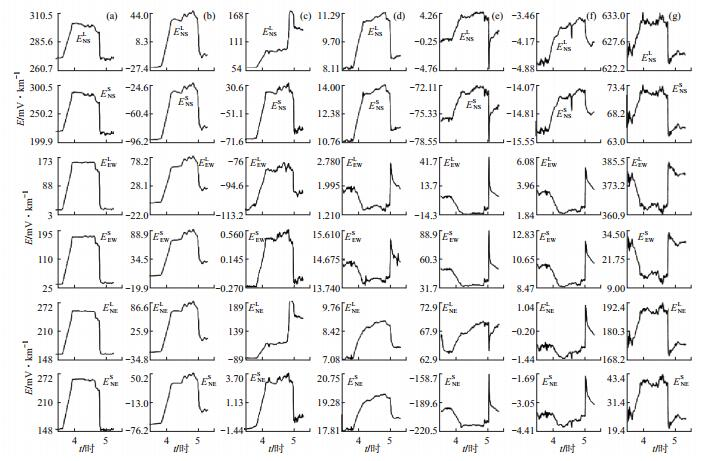图 17 2013年7月25日华东地区部分地电场台站受直流输电干扰波形（据马钦忠等（2014）） (a)青浦；(b)崇明；(c)长江农场；(d)海安；(e)南京；(f)高邮；(g)嘉山 Fig.17 The HVDC disturbance waveform observated by the partial geoelectric field stations in East China on July 25, 2013 (Ma Qinzhong et al, 2014)
4.2.4 工频干扰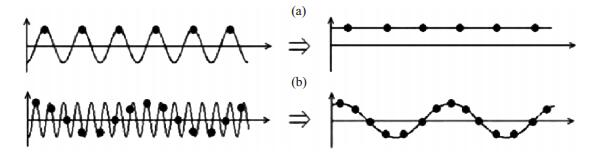图 18 工频干扰频率混叠效应原理示意 (a)直流干扰；(b)低频干扰 Fig.18 The principle of the power frequency interference generated by the frequency aliasing effect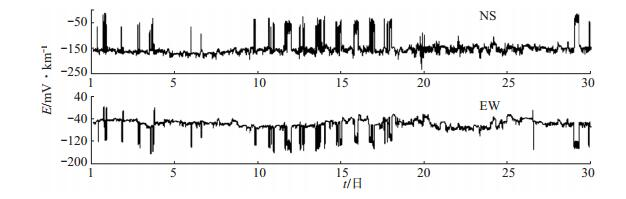图 19 2007年11月河北省赤城地震台地电场观测分钟数据曲线 Fig.19 The minute data curve of the geoelectric field observated by Chicheng Seismic Station, Hebei Province, during November 2007
4.3 大地震前后地电场变化

2006年7月4日河北文安县发生MS 5.1地震，马钦忠（2008）利用地电场多极距观测系统噪音识别原理及其在地电场数据分析中的应用方法，通过对首都圈6个地电场台观测资料的研究分析，逐一排查所选众多震前地电场异常信号，基本认定其中5组为震前地电场异常变化信号。宝抵台2006年6月26日、27日地电场观测结果为其中1组异常信号，见图 20。对认定的5组震前异常信号分析表明：①文安MS 5.1地震前存在地电场异常变化信号；②文安MS 5.1地震前地电场异常变化信号的出现存在“敏感点”效应；③地电场异常变化信号在不同台站具有不同步现象，且异常结束至发震时间间隔不同；④距震中区较近的宝坻台记录的震前地电场异常幅度较小，距震中区较远的昌黎台记录的震前地电场异常幅度则较大。上述事实表明，地电场异常变化信号的出现存在某种程度的“敏感点”效应，其可能的物理解释为：不同测点地下电性结构的差异性，是导致地电场信号差异的基本控制因素；地电场的异常变化与测点下方介质的电阻率异常变化相关联，也可能导致地电场异常的差异性。也就是说，把地电场观测与台站基础电性结构联系起来，是推进地电场观测并发挥其在地震预测中效能的重要环节之一。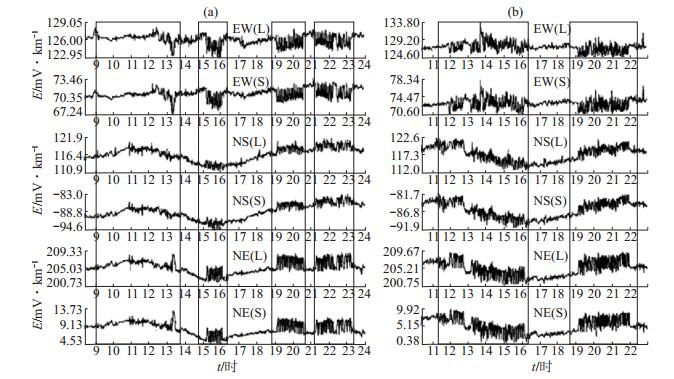图 20 宝抵台2006年6月26日、27日观测结果鉴别的自然电场异常信号（据马钦忠（2008）） (a)2006年6月26日；(b)2006年6月27日 Fig.20 The anomalous signals of the spontaneous potential observated and identified by Baodai station on June 26 and 27, 2006 (Ma Qinzhong, 2008)

2015年4月25日尼泊尔8.1级特大地震前后，在距震中630 km的中国西藏自治区拉萨地磁台，记录到突出的地电场扰动变化，见图 21。该地电场扰动变化的各分量峰值均超过1 000 mV/km，时间跨度达20个月以上。鉴于该组数据变化的典型性，席继楼等（2016）就尼泊尔MS 8.1特大地震前后拉萨台地电场观测数据异常变化的分析研究结果，在“亚洲大洋洲地球科学学会2016年度学术大会（AOGS2016）”上进行了交流讨论，得到国内外学者的较大关注。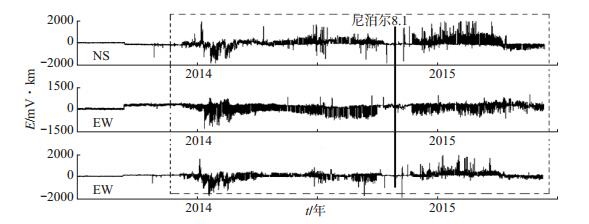图 21 尼泊尔MS 8.1地震前后拉萨台地电场观测分钟数据曲线 Fig.21 The minute data curve of the geoelectric field observated by Lhasa station before and after Nepal MS 8.1 earthquake
5 总结与展望

 邓明, 刘志刚, 白宜诚, 等. 海底电场传感器原理及研制技术[J]. 地质与勘探, 2001, 38(6): 43-47. 董颂声, 陆学振, 冯志生, 等. 希腊VAN地震预报方法的进展与争论(Ⅱ)--国际评价与争论[J]. 国际地震动态, 1999(1): 12-21. DOI:10.3969/j.issn.0253-4975.1999.01.003 冯志生, 董颂声, 王建宇, 等. 希腊VAN地震预报方法的进展与争论(Ⅰ)--进展[J]. 国际地震动态, 1998(12): 11-17. 傅承义, 陈运泰, 祁贵仲. 地球物理学基础[M]. 北京: 科学出版社, 1985. 郝建国, 潘怀文, 毛国敏, 等. 准静电场异常与地震--一种可靠短临地震前兆信息探索[J]. 地震地磁观测与研究, 2000, 21(4): 3-7. DOI:10.3969/j.issn.1003-3246.2000.04.001 胡明朝, 席继楼, 刘超, 等. 地电场观测的低功耗技术研究[J]. 地震地磁观测与研究, 2011, 32(1): 71-76. DOI:10.3969/j.issn.1003-3246.2011.01.013 黄清华. 地震电磁观测研究简述[J]. 国际地震动态, 2005(11): 2-5. DOI:10.3969/j.issn.0253-4975.2005.11.002 黄清华.地震电磁现象的物理学研究[C]//中国地球物理学会第二十七届年会论文集.长沙: 中国地球物理年会, 2011. 姜振海, 石航, 史小平. 固体不极化电极现场实验研究[J]. 地震研究, 2004, 27(Z1): 57-62. DOI:10.3969/j.issn.1000-0666.2004.z1.012 李宁.天祝地震空区地震电磁前兆现象研究[D].兰州: 中国地震局兰州地震研究所, 2007: 1-59. 刘国栋, 陈乐寿. 大地电磁测深研究[M]. 北京: 地震出版社, 1984. 刘君, 章鑫, 杜学彬, 等. 青藏高原东北缘区域地电脉动能谱变化分析[J]. 地震学报, 2017, 39(4): 579-592. 马钦忠. 希腊"VAN"方法地电流预报地震介绍[J]. 防灾博览(地震科技情报), 1997(1): 5-10. 马钦忠. 地电场多极距观测装置系统与文安MS 5.1地震前首都圈地电场异常研究[J]. 地震学报, 2008, 30(6): 615-625. DOI:10.3321/j.issn:0253-3782.2008.06.007 马钦忠, 唐玉雄, 张永仙, 等. 2008年西藏4次MS 6.0以上地震前拉萨地电场异常信号特征[J]. 地震, 2011, 31(1): 86-97. 马钦忠, 李伟, 张继红, 等. 与大电流信号有关的华北东部地区地电场空间变化特征的研究[J]. 地球物理学报, 2014, 57(2): 518-530. 钱家栋, 杨冬梅, 熊仲华, 等. 地震电磁学理论基础与观测技术[M]. 北京: 地震出版社, 2010. 宋艳茹, 席继楼, 刘超, 等. 一种Pb-PbCl2不极化电极试验研究[J]. 地震地磁观测与研究, 2011, 32(6): 97-103. DOI:10.3969/j.issn.1003-3246.2011.06.017 孙君嵩, 杜学彬. 中国大陆地电暴时频特征[J]. 地震学报, 2017, 39(4): 615-632. 孙正江, 王华俊. 地电概论[M]. 北京: 地震出版社, 1984. 谭大诚, 赵家骝, 席继楼, 等. 潮汐地电场特征及机理研究[J]. 地球物理学报, 2010, 53(3): 544-555. 谭大诚, 王兰炜, 赵家骝, 等. 潮汐地电场谐波和各向波形的影响要素[J]. 地球物理学报, 2011, 54(7): 1842-1853. DOI:10.3969/j.issn.0001-5733.2011.07.018 谭大诚, 赵家骝, 席继楼, 等. 青藏高原中强地震前的地电场变异及构成解析[J]. 地球物理学报, 2012, 55(3): 875-885. DOI:10.6038/j.issn.0001-5733.2012.03.017 谭大诚, 席继楼, 张慧, 等. 地电场水文地质因素及裂隙水主体渗流方向逐日计算[J]. 地震学报, 2013, 35(1): 36-49. DOI:10.3969/j.issn.0253-3782.2013.01.005 谭大诚, 赵家骝, 刘小凤, 等. 自然电场的区域性变化特征[J]. 地球物理学报, 2014, 57(5): 1588-1598. 田山, 王建国, 徐学恭, 等. 大地电场观测地震前兆异常提取技术研究[J]. 地震学报, 2009, 31(4): 424-431. DOI:10.3321/j.issn:0253-3782.2009.04.008 田山, 张磊, 王建国, 等. 汶川、玉树大地震前的地电场异常[J]. 地球物理学进展, 2012, 27(3): 878-887. 王洪亮.高压直流输电单极大地回线方式运行时接地极电流的研究[D].成都: 西南交通大学, 2007: 1-42. 卫云鸽, 曹全喜, 黄云霞, 等. 海洋电场传感器低噪声Ag/AgCl电极的制备及性能[J]. 人工晶体学报, 2009, 38(Z1): 394-398. 席继楼, 赵家骝, 王燕琼, 等. 地电场观测技术研究[J]. 地震, 2002, 22(2): 67-73. 席继楼, 米玛, 旦增, 等. 当雄MS 6.6地震前后地电场观测数据分析研究[J]. 地震地磁观测与研究, 2009, 30(Z1): 8-15. 席继楼, 宋艳茹, 胡明朝, 等. 全方位自然电场观测方法和观测技术研究[J]. 地震学报, 2013, 35(1): 94-107. DOI:10.3969/j.issn.0253-3782.2013.01.010 席继楼, 赵家骝, 关歆莹, 等. 地电场观测中的工频干扰抑制方法研究[J]. 地震, 2015, 35(4): 53-63. 席继楼, 关华平, 刘超, 等. 2015年尼泊尔8.1级地震前后拉萨地电场观测数据变化分析[J]. 地震, 2016, 36(2): 1-13. 席继楼, 赵家骝, 刘超, 等. 新型网络化地电场观测技术研究与应用[J]. 地球物理学进展, 2016, 31(6): 2690-2699. 席继楼, 关华平, 刘超, 等. 几次大地震前后地电场中长期变化分析与研究[J]. 地震, 2018, 38(2): 117-126. DOI:10.3969/j.issn.1000-3274.2018.02.011 席继楼, 庄楠, 刘超, 等. 自然电场全方位观测试验与地表影响因素分析研究[J]. 地震, 2018, 38(4): 49-61. 叶青, 杜学彬, 周克昌, 等. 大地电场变化的频谱特征[J]. 地震学报, 2007, 29(4): 382-390. DOI:10.3321/j.issn:0253-3782.2007.04.005 张颖, 席继楼. 区域地电场观测数据分析研究[J]. 地震地磁观测与研究, 2008, 29(3): 29-34. DOI:10.3969/j.issn.1003-3246.2008.03.005 赵家骝, 苏明达, 王燕琼, 等. ZD9大地电场仪[J]. 西北地震学报, 1995, 17(1): 69-74. 庄楠, 席继楼, 刘超, 等.地电场观测中的地铁干扰特征分析讨论[C]//中国地震学会地震电磁学专业委员会2013年年会论文集.银川: 中国地震学会地震电磁学专业委员会, 2013. [美]考夫曼, 凯勒.地电磁测深法[M].刘国栋, 赵国泽, 译.北京: 地震出版社, 1987. Ifantis A & Giannakopoulos K. New experimental data reveal possible relation of chaotic behavior of the long-term geoelectric potential difference to seismic activity in Western Greece[J]. Chaos, Solitons & Fractals, 2007, 34(3): 717-726. Orihara Y, Kamogawa M, Nagao T, et al. Independent component analysis of geoelectric field data in the northern Nagano, Japan[J]. Proceedings of the Japan Academy, Series B, 2009, 85(9): 435-442. DOI:10.2183/pjab.85.435 Sobolev G A. Application of electric method to the tentative short-term forecast of Kamchatka earthquakes[J]. Pure & Applied Geophysics, 1975, 113(1): 229-235. Uyeda S, Hayakawa M, Nagao T, et al. Electric and magnetic phenomena observed before the volcano-seismic activity in 2000 in the Izu Island Region, Japan[J]. Proceedings of the National Academy of Sciences of the United States of America, 2002, 99(11): 7352-7355. DOI:10.1073/pnas.072208499 Uyeda S, Nagao T, Orihara Y, et al. Geoelectric potential changes: Possible precursors to earthquakes in Japan[J]. Proceedings of the National Academy of Science of the United States of America, 2000, 97(9): 4561-4566. DOI:10.1073/pnas.97.9.4561 Uyeda S, Nagao T, Hattori K, et al. Geophysical observatory in Kamchatka region for monitoring of phenomena connected with seismic activity[J]. Natural Hazards and Earth System Sciences, 2001, 1(1/2): 3-7. DOI:10.5194/nhess-1-3-2001 Varotsos P, Alexopoulos K, Nomicos K, et al. Official earthquake prediction procedure in Greece[J]. Tectonophysics, 1988, 152(3/4): 193-196. Varotsos P A, Sarlis N V, Skordas E S. Long-range correlations in the electric signals that precede rupture: Further investigations[J]. Physical Review E, 2003, 67(2): 021109 DOI:10.1103/PhysRevE.67.021109